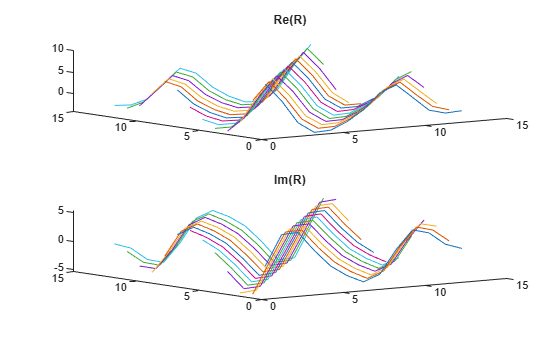Main Content

# corrmtx

Data matrix for autocorrelation matrix estimation

## Syntax

``H = corrmtx(x,m)``
``H = corrmtx(x,m,method)``
``[H,r] = corrmtx(___)``

## Description

````H = corrmtx(x,m)` returns an (n+`m`)-by-(`m`+1) rectangular Toeplitz matrix H = `H` such that H†H is a biased estimate of the autocorrelation matrix for the input vector `x`. n is the length of `x`, `m` is the prediction model order, and H† is the conjugate transpose of H.```

example

````H = corrmtx(x,m,method)` computes the matrix `H` according to the method specified by `method`.```
````[H,r] = corrmtx(___)` also returns the (`m` + 1)-by-(`m` + 1) autocorrelation matrix estimate `r`, computed as H†H, for any of the previous syntaxes.```

## Examples

collapse all

Generate a signal composed of three complex exponentials embedded in white Gaussian noise. Compute the data and autocorrelation matrices using the `'modified'` method.

```n = 0:99; s = exp(i*pi/2*n)+2*exp(i*pi/4*n)+exp(i*pi/3*n)+randn(1,100); m = 12; [X,R] = corrmtx(s,m,'modified');```

Plot the real and imaginary parts of the autocorrelation matrix.

```[A,B] = ndgrid(1:m+1); subplot(2,1,1) plot3(A,B,real(R)) title('Re(R)') subplot(2,1,2) plot3(A,B,imag(R)) title('Im(R)')```## Input Arguments

collapse all

Input data, specified as a vector.

Prediction model order, specified as a positive real integer.

Matrix computation method, specified as `'autocorrelation'`, `'prewindowed'`, `'postwindowed'`, `'covariance'` or `'modified'`.

• `'autocorrelation'`: (default) `H` is the (n + `m`)-by-(`m` + 1) rectangular Toeplitz matrix that generates an autocorrelation estimate for the length-n data vector `x`, derived using prewindowed and postwindowed data, based on an `m`th-order prediction model. The matrix can be used to perform autoregressive parameter estimation using the Yule-Walker method. For more details, see `aryule`.

• `'prewindowed'`: `H` is the n-by-(`m` + 1) rectangular Toeplitz matrix that generates an autocorrelation estimate for the length-n data vector `x`, derived using prewindowed data, based on an `m`th-order prediction model.

• `'postwindowed'`: `H` is the n-by-(`m` + 1) rectangular Toeplitz matrix that generates an autocorrelation estimate for the length-n data vector `x`, derived using postwindowed data, based on an `m`th-order prediction model.

• `'covariance'`: `H` is the (n`m`)-by-(`m` + 1) rectangular Toeplitz matrix that generates an autocorrelation estimate for the length-n data vector `x`, derived using nonwindowed data, based on an `m`th-order prediction model. The matrix can be used to perform autoregressive parameter estimation using the covariance method. For more details, see `arcov`.

• `'modified'`: `H` is the 2(n`m`)-by-(`m` + 1) modified rectangular Toeplitz matrix that generates an autocorrelation estimate for the length-n data vector `x`, derived using forward and backward prediction error estimates, based on an `m`th-order prediction model. The matrix can be used to perform autoregressive parameter estimation using the modified covariance method. For more details, see `armcov`.

## Output Arguments

collapse all

Data matrix, returned for autocorrelation matrix estimation. The size of `H` depends on the matrix computation method specified in `method`.

Biased autocorrelation matrix, returned as a (`m` + 1)-by-(`m` + 1) rectangular Toeplitz matrix.

## Algorithms

The Toeplitz data matrix computed by `corrmtx` depends on the method you select. The matrix determined by the autocorrelation (default) method is:

`$H=\frac{1}{\sqrt{n}}\left[\begin{array}{ccccc}x\left(1\right)& 0& \cdots & 0& 0\\ x\left(2\right)& x\left(1\right)& \cdots & 0& 0\\ x\left(3\right)& x\left(2\right)& \cdots & 0& 0\\ ⋮& ⋮& \ddots & ⋮& ⋮\\ x\left(m\right)& x\left(m-1\right)& \cdots & x\left(1\right)& 0\\ x\left(m+1\right)& x\left(m\right)& \cdots & x\left(2\right)& x\left(1\right)\\ x\left(m+2\right)& x\left(m+1\right)& \cdots & x\left(3\right)& x\left(2\right)\\ ⋮& ⋮& \ddots & ⋮& ⋮\\ x\left(n-1\right)& x\left(n-2\right)& \cdots & x\left(n-m\right)& x\left(n-m-1\right)\\ x\left(n\right)& x\left(n-1\right)& \cdots & x\left(n-m+1\right)& x\left(n-m\right)\\ 0& x\left(n\right)& \cdots & x\left(n-m+2\right)& x\left(n-m+1\right)\\ ⋮& ⋮& ⋰& ⋮& ⋮\\ 0& 0& \cdots & x\left(n-1\right)& x\left(n-2\right)\\ 0& 0& \cdots & x\left(n\right)& x\left(n-1\right)\\ 0& 0& \cdots & 0& x\left(n\right)\end{array}\right].$`

In the matrix, m is the same as the input argument `m` to `corrmtx` and n is `length(x)`. Variations of this matrix are used to return the output `H` of `corrmtx` for each method:

• `'autocorrelation'` — (default) `H` = H.

• `'prewindowed'``H` is the n-by-(m + 1) submatrix of H whose first row is [x(1) … 0] and whose last row is [x(n) … x(nm)].

• `'postwindowed'``H` is the n-by-(m + 1) submatrix of H whose first row is [x(m + 1) … x(1)] and whose last row is [0 … x(n)].

• `'covariance'``H` is the (nm)-by-(m + 1) submatrix of H whose first row is [x(m + 1) … x(1)] and whose last row is [x(n) … x(nm)].

• `'modified'``H` is the 2(nm)-by-(m + 1) matrix Hmod defined by

`${H}_{\mathrm{mod}}=\frac{1}{\sqrt{2\left(n-m\right)}}\left[\begin{array}{ccc}x\left(m+1\right)& \cdots & x\left(1\right)\\ ⋮& ⋰& ⋮\\ x\left(n\right)& \cdots & x\left(n-m\right)\\ {x}^{\ast }\left(1\right)& \cdots & {x}^{\ast }\left(m+1\right)\\ ⋮& \ddots & ⋮\\ {x}^{\ast }\left(n-m\right)& \cdots & {x}^{\ast }\left(n\right)\end{array}\right].$`

 Marple, S. Lawrence. Digital Spectral Analysis: With Applications. Prentice-Hall Signal Processing Series. Englewood Cliffs, N.J: Prentice-Hall, 1987.

## Support

#### Deep Learning for Signal Processing with MATLAB

Download white paper